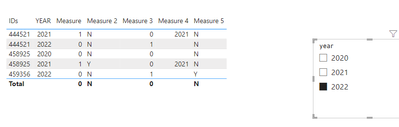cancel
Showing results for
Did you mean:Super User

## Row Delta between two years (actual and previous)

I try to explain it to you simply. I have one column with user IDs and a second column with the year that user is active, like this:

 IDs YEAR FLAG AAA 2021 N AAA 2022 N BBB 2021 Y

I want to calculate a dynamic Flag that indicates the users who were active the year before the selected one but who are no longer active in the selected one.

By selecting 2022 for example, the user AAA is flagged on N, as he was active both in 2021 and in 2022. The user BBB, on the other hand, will be flagged with Y, as he was active in 2021, the year previous to the filtered one and no longer present in the 2022.

Therefore in visualization we will have a table that must be filtered by the Flag (column or measure) without the Flag field being present in the table and a visual filter on the year.
I emphasize that it must be dynamic, in the sense that by filtering 2022, the comparison must only be on 2021, 2020 must not be taken into consideration.

I am attaching a pbix with an example dataset. In the pbix, filtering on 2022, the only ID to appear must be 458925.

Thx u,

BBF

1 ACCEPTED SOLUTIONCommunity Support

Hi @BeaBF ,

Plaese refer to my pbix file to see if it helps you.

Create another table with year column. Then put the column into the slicer.

Then create  measures.

``````Measure = var _1=SELECTEDVALUE('Table (2)'[year])
var _1re=
IF(_1-1=SELECTEDVALUE('Table'[YEAR]),1,0)
return _1re``````
``````Measure 2 = var _1= IF([Measure]=1,0,1)
var _2=CALCULATE(MAX('Table'[IDs]),FILTER(ALL('Table'),'Table'[IDs]=SELECTEDVALUE('Table'[IDs])&&'Table'[YEAR]=SELECTEDVALUE('Table (2)'[year])))
return
IF([Measure]=1&&_2=BLANK(),"Y","N")``````If it does not help, please provide more details with your desired output and pbix file without privacy information (or some sample data) .

Best Regards
Community Support Team _ Polly

If this post helps, then please consider Accept it as the solution to help the other members find it more quickly.

5 REPLIES 5Community Support

Hi @BeaBF ,

Plaese refer to my pbix file to see if it helps you.

Create another table with year column. Then put the column into the slicer.

Then create  measures.

``````Measure = var _1=SELECTEDVALUE('Table (2)'[year])
var _1re=
IF(_1-1=SELECTEDVALUE('Table'[YEAR]),1,0)
return _1re``````
``````Measure 2 = var _1= IF([Measure]=1,0,1)
var _2=CALCULATE(MAX('Table'[IDs]),FILTER(ALL('Table'),'Table'[IDs]=SELECTEDVALUE('Table'[IDs])&&'Table'[YEAR]=SELECTEDVALUE('Table (2)'[year])))
return
IF([Measure]=1&&_2=BLANK(),"Y","N")``````If it does not help, please provide more details with your desired output and pbix file without privacy information (or some sample data) .

Best Regards
Community Support Team _ Polly

If this post helps, then please consider Accept it as the solution to help the other members find it more quickly.Super User

It's perfect! I would have one more step, calculate the same Flag for the IDs which are present in the current year of selection (2022 for example) and which weren't there in the previous year (2021 if 2022 is selected). Could you help me with this new piece?

In the pbix, filtering on 2022, only ID 459356 should be flagged to Y, as it is not present in 2021. Filtering on 2021, instead, only ID 444521 should be set to Y, as it is not present in 2020.

Thank you very much,

BBFCommunity Support

Hi @BeaBF ,

Please refer to my pbix file.

``````Measure 3 = var _1=SELECTEDVALUE('Table (2)'[year])
var _1re=
IF(_1=SELECTEDVALUE('Table'[YEAR]),1,0)
return _1re``````
``````Measure 4 = var _1= IF([Measure 3]=1,0,1)
var _2=CALCULATE(MAX('Table'[IDs]),FILTER(ALL('Table'),'Table'[IDs]=SELECTEDVALUE('Table'[IDs])&&'Table'[YEAR]=SELECTEDVALUE('Table (2)'[year])))
var _1year=SELECTEDVALUE('Table (2)'[year])-1
var _1re=CALCULATE(MAX('Table'[YEAR]),FILTER(ALL('Table'),'Table'[YEAR]=_1year))
var _1jie=IF(MAX('Table'[YEAR])=_1re,_1re,BLANK())
var _111=MAXX(FILTER(ALL('Table'),'Table'[IDs]=SELECTEDVALUE('Table'[IDs])),_1jie)
return _111
//IF([Measure 3]=1&&_2=BLANK(),"Y","N")``````
``````Measure 5 = var _1=MAXX(FILTER(ALL('Table'),'Table'[IDs]=SELECTEDVALUE('Table'[IDs])),[Measure 4])
return
IF(_1=BLANK()&&[Measure 3]=1,"Y","N")``````Best Regards
Community Support Team _ Polly

If this post helps, then please consider Accept it as the solution to help the other members find it more quickly.Super User

@v-rongtiep-msft I created these two measures:

Measure 3 = var _1=SELECTEDVALUE('Table (2)'[year])
var _1re=
IF(_1=SELECTEDVALUE('Table'[YEAR]),1,0)
return _1re

Measure 4 = var _1= IF([Measure 3]=1,0,1)
var _2=CALCULATE(MAX('Table'[IDs]),FILTER(ALL('Table'),'Table'[IDs]=SELECTEDVALUE('Table'[IDs])&&'Table'[YEAR]=SELECTEDVALUE('Table (2)'[year]) - 1))
return
IF([Measure 3]=1&&_2=BLANK(),"Y","N")

Do they look correct to you?

BBFCommunity Support

Hi @BeaBF ,

Yours seems a little simpler.

Best Regards
Community Support Team _ Polly

If this post helps, then please consider Accept it as the solution to help the other members find it more quickly.Announcements#### Exclusive opportunity for Women!

Join us for a free, hands-on Microsoft workshop led by women trainers for women where you will learn how to build a Dashboard in a Day!#### Power Platform Conference-Power BI and Fabric Sessions

Join us Oct 1 - 6 in Las Vegas for the Microsoft Power Platform Conference.Top Solution Authors
Top Kudoed Authors
Users online (1,870)